## Solutions of Refraction of Light Lakhmir Singh Manjit Kaur VSAQ, MCQ, HOTS, and LAQ Pg No. 219 Class 10 Physics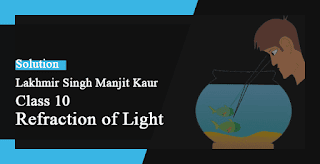1. If a ray of light goes from a rarer medium to a denser medium, will it bend towards the normal or away from it?

→ If a ray of light goes from a rarer medium to a denser medium, It will bend towards the normal.

2. If a ray of light goes from a denser medium to a rarer medium, will it bend towards the normal or away from the normal?

→ If a ray of light goes from a denser medium to a rarer medium, It will bend away from the normal.

3. A beam of light travelling in a rectangular glass slab emerges into air. Draw a ray-diagram indicating the change in its path.

→ A beam of light travelling in a rectangular glass slab emerges into air. The ray diagram indicating its path is as shown.4. A beam of light travelling in air is incident on water. Draw a ray-diagram indicating the change in its path in water.

→ A beam of light travelling in air is incident on water. the ray-diagram indicating the change in its path in water is5. A ray of light travelling in water emerges into air. Draw a ray-diagram indicating the change in its path.

→ A ray of light travelling in water emerges into air. The ray-diagram indicating the change in its path is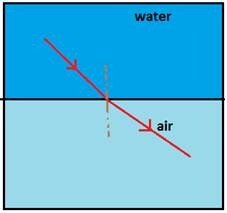6. A ray of light travelling in air is incident on a parallel-sided glass slab (or rectangular glass slab). Draw a ray-diagram indicating the change in its path in glass.

→ A ray of light travelling in air is incident on a parallel-sided glass slab (or rectangular glass slab). The ray-diagram indicating the change in its path in glass is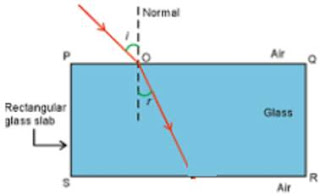7. A ray of light travelling in glass emerges into air. State whether it will bend towards the normal or away from the normal.

→ A ray of light travelling in glass emerges into air. The ray of light will bend away from the normal.

8. A ray of light travelling in air enters obliquely into water. Does the ray of light bend towards the normal or away from the normal? Why?

→ The ray of light bends towards the normal. This is because water is an optically denser medium than air.

9. A ray of light goes from water into air. Will it bend towards the normal or away from the normal?

→ A ray of light goes from water into air. It will bend away from the normal.10. State two effects caused by the refraction of light.

→ Two effects caused by refraction of light are:
1) A swimming pool always looks shallower than it really is, because the light coming from the bottom of the pool bends when it comes out at the surface due to refraction of light.2) A straight stick which is immersed partly in water always looks to be bent at the surface of water, because the light coming from the stick bends when it comes out at the surface due to refraction of light.11. Name the phenomenon due to which a swimming pool appears less deep than it really is.

→ The phenomenon due to which a swimming pool appears less deep than it really is due to refraction of light.

12. When a ray of light passes from air into glass, is the angle of refraction greater than or less than the angle of incidence?

→ When a ray of light passes from air into glass Angle of refraction is less than the angle of incidence.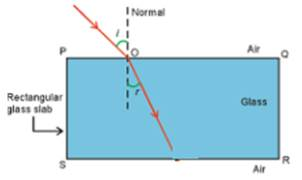13. A ray of light passes from air into a block of glass. Does it bend towards the normal or away from it?

→ A ray of light travelling from air to glass block, will bend towards the normal.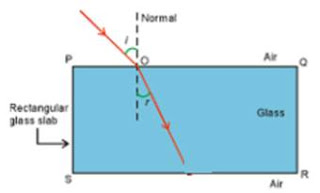14. As light rays pass from water into glass, are they refracted towards the normal or away from the normal?

→ A ray of light travelling from water into glass will bend towards the normal.15. In which material do you think light rays travel faster-glass or air?

→ Air.

16. Which phenomenon of light makes the water to appear shallower than it really is?

→ Refraction of light makes the water to appear shallower than it really is.

17. State whether the following statement is true or false: Refraction occurs because light speeds up in denser materials.

→ False.

18. Why does a ray of light bend when it travels from one medium to another?

→ Due to the change in the speed of light.

19. Fill in the following blanks with suitable words:
(a) Light travelling along a ____ is not refracted.
(b) Light bends when it passes from water into air. We say that it is _____

→ (a) normal
(b) refracted

20. What is meant by ‘refraction of light’? Draw a labeled ray diagram to show the refraction of light.

→ Refraction of light is the change in the direction of light when it passes from one medium to another.21. A ray of light travelling in air is incident on a rectangular glass block and emerges out into the air from the opposite face. Draw a labeled ray diagram to show the complete path of this ray of light. Mark the two points where the refraction of light takes place. What can you say about the final direction of ray of light?A ray of light travelling in air is incident on a rectangular glass block and emerges out into the air from the opposite face. We can say about the final direction of the ray of light is that it is same as the incident direction.

22. Draw a labeled ray diagram to show how a ray of light is refracted when it passes:
(a) From air into an optically denser medium.
(b) From an optically denser medium into air.

→ (a) Ray of light travelling from air into an optically denser medium (water).(b) Ray of light travelling from an optically denser medium(water) into air.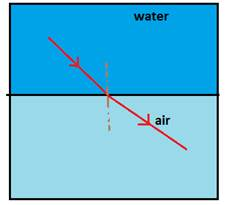23. The diagram given alongside shows a ray of light entering a rectangular block of glass.
(a) Copy the diagram and draw the normal at the point of entry.
(b) Draw the approximate path of the ray of light through the glass block and out of the other side.

→ (a) a ray of light entering a rectangular block of glass will have normal as seen(b) approximate path of the ray of light through the glass block and out of the other side is24. What is meant by the 'angle of incidence' and the 'angle of refraction' for a ray of light? Draw a labeled ray diagram to show the angle of incidence and the angle of refraction for a refracted ray of light.

→ Angle of incidence is the angle between the incident ray and normal at the point of incidence.
Angle of refraction is the angle between the refracted ray and normal at the point of refraction.
A labeled ray diagram to show the angle of incidence and the angle of refraction for a refracted ray of light is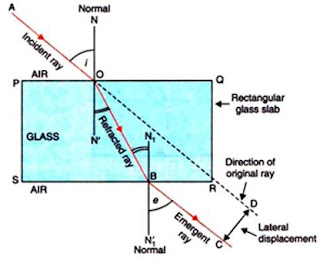25. Light travels more quickly through water than through glass.
(a) Which is optically denser: water or glass?
(b) If a ray of light passes from glass into water, which way will it bend: towards the normal or away from the normal?

→ (a) Light travels more quickly through water than through glass as Glass is optically denser than the water.(b) If a ray of light passes from glass into water then the ray will bend away from the normal.26. Draw a labeled ray diagram to show how a ray of light passes through a parallel sided glass block
(a) If it hits the glass block at 90° (that is, perpendicular to the glass block)
(b) If it hits the glass block at an angle other than 90° (that is, obliquely to the glass block).

→ (a) a labeled ray diagram of a ray of light that passes through a parallel sided glass block, if it hits the glass block at 90°.(b)a labeled ray diagram of a ray of light that passes through a parallel sided glass block, if ray of light hits the block other than the  90°.27. When a light ray passes from air into glass, what happens to its speed? Draw a diagram to show which way the ray of light bends.

→ When a light ray passes from air into glass, the speed of the ray decreases.The ray of light bends towards the normal.

28A. Explain why, a stick half immersed in water appears to be bent at the surface. Draw a labeled diagram to illustrate your answer.

→ A straight stick which is immersed partly in water always looks to be bent at the surface of water, because the light coming from the stick bends when it comes out at the surface due to refraction of light.28B. A coin in a glass tumbler appears to rise as the glass tumbler is slowly filled with water. Name the phenomenon responsible for this effect.

→ A coin or stone lying at the bottom of a container filled with water appears to be raised because of refraction of light.29A. With the help of a labeled diagram, explain why a tank full of water appears less deep than it actually is.

→ If we look into a tank of water, it appears to be less deep than it really is because the refraction of light causes us to see a virtual image of the bottom of the pool coming from the water into the air.

29B. Name the phenomenon due to which a pencil partly immersed in water and held obliquely appears to be bent at the water surface.

→ The phenomenon due to which a pencil partly immersed in water and held obliquely appears to be bent at the water surface is Refraction of light.

30A. With the help of a diagram, show how when light falls obliquely on the side of a rectangular glass slab, the emergent ray is parallel to the incident ray.

→ When light falls obliquely on the side of a rectangular glass slab, the emergent ray is parallel to the incident ray.30B. Show the lateral displacement of the ray on the diagram.

→ The lateral displacement is shown by ‘d’.30C. State two factors on which the lateral displacement of the emergent ray depends.

→ Factors on which the lateral displacement depends are:
(i) Angle of incidence
(ii) Thickness of glass slab
(iii) Refractive index of glass slab

31. Explain with the help of a labeled ray diagram, why a pencil partly immersed in water appears to be bent at the water surface. State whether the bending of pencil will increase or decrease if water is replaced by another liquid which is optically more dense than water. Give reason for your answer.

→ The labeled ray diagram of a pencil partly immersed in water appears to be bent at the water surface.The bending of pencil will increase decrease if water is replaced by another liquid which is optically more dense than water. The optically denser medium will cause more refraction (or more bending) of light rays.

Multiple Choice Questions (MCQs)-Pg-221

32. Light travelling from a denser medium to a rarer medium along a normal to the boundary:
A. Is refracted towards the normal
B. Is refracted away from the normal
C. Goes along the boundary
D. Is not refracted

→ Light travelling from a denser medium to a rarer medium along a normal to the boundary Is not refracted.33. A ray of light passes from glass into air. The angle of refraction will be:
A. Equal to the angle of incidence
B. greater than the angle of incidence
C. Smaller than the angle of incidence
D. 45°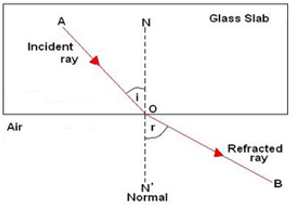34. A ray of light travelling in air goes into water. The angle of refraction will be:
A. 90°
B. Smaller than the angle of incidence
C. Equal to the angle of incidence
D. Greater than the angle of incidence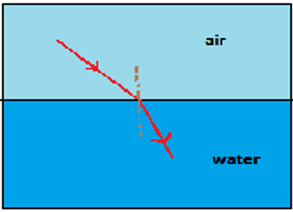35. The speed of light in air is:
A. 3 x 108 cm/s
B. 3 x 108 km/s
C. 3 x 108 mm/s
D. 3 x 108 m/s

→ The speed of light in air is 3 x 108 m/s.

36. When a ray of light travelling in glass enters into water obliquely:
A. It is refracted towards the normal
B. It is not refracted at all
C. It goes along the normal
D. It is refracted away from the normal37. A ray of light travelling in water falls at right angles to the boundary of a parallel-sided glass block. The ray of light:
A. Is refracted towards the normal
B. Is refracted away from the normal
C. Does not get refracted
D. Is reflected along the same path.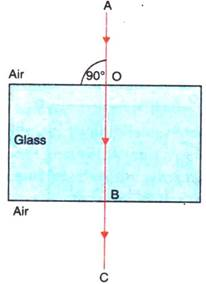38. A ray of light passes from a medium X to another medium Y. No refraction of light occurs if the ray of light hits the boundary of medium Y at an angle of:
A. 0°
B. 45°
C. 90°
D. 120°Questions Based on High Order Thinking Skills (HOTS)-Pg-221

39. Which of the following diagrams shows the ray of light refracted correctly?→ (A)

40. A vertical ray of light strikes the horizontal surface of some water:
(a) What is the angle of incidence?
(b) What is the angle of refraction?

→ (a) the angle of incidence is 0o
(b) the angle of refraction is 0o

41. How is the reflection of light ray from a plane mirror different from the refraction of light ray as it enters a block of glass?

→ The reflection of light ray from a plane mirror different from the refraction of light ray as when a ray of light enters a block of plane mirror the angle of reflection is equal to the angle of incidence but the angle of refraction is not equal to the angle of incidence when it enters a block of glass.

42. How does the light have to enter the glass?
(a) To produce a large amount of bending?
(b) For no refraction to happen?

→ (a) the light have to enter the glass Obliquely To produce a large amount of angle of incidence.
(b) the light have to enter the glass perpendicularly (at right angles) to the glass surface For no refraction to happen.

43A. How can you bend light away from the normal?

→ We can bend light away from the normal by making the light enter from a denser medium to a rarer medium.

43B. How must light travel out of a substance if it is not going to be refracted?